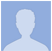## Probability Jam

### Rack using the Clock Multiplier and Gate Probability blocks

David X
1.0 (Updated 3 months ago)
126.4kB
April 11, 2020
Reaktor 6
Blocks Racks

#### DESCRIPTION

An example of using the Clock Multiplier and Gate Probability blocks (This rack requires the toybox Synth Pack).

This is how it works:

• A 16th note clock signal is sent to the 4 channels of the ‘Clock Multiplier’ block. The ‘Clock Multiplier’ multiplies and divides the incoming clock into 8th notes, 1/4 notes etc.

• The 4 clock signals then pass into the ‘Gate Probability’ block and this thins out the clock signals according the value of the knobs: if the knobs are at 100 then there is a 100% probability that the clock will be allowed through, as the knob is decreased it becomes less and less likely the incoming clocks will be passed through to the block's output.

• The gates are then passed to 4 ‘Mini Synth’ blocks. These are set up with 4 different sounds.

• The pitch of the first Mini Synth is controlled by a ‘Randomizer’ block triggered by the gate signal. This block creates random values. The ‘Randomizer’ block is connected to the ‘1 OCTAVE’ port of the ‘Transpose’ block. This port limits the outputted pitch signals to just a single octave. The pitch is also snapped to a pentatonic scale using the ‘SCALE’ knob.

• The 4 Mini Synth blocks are mixed together using a mixer block, a separate mixer block is used as a ‘reverb send'.

• The mixer and reverb signals are summed before being passed to Reaktor’s output.

• There are also a couple of LFOs that modulate the 'MULTIPLY' knobs of the ‘Clock Multiplier’ to create rhythmic variations.

Dependancies:

toybox Synth Pack